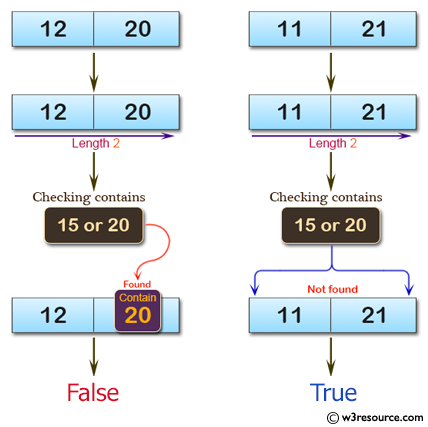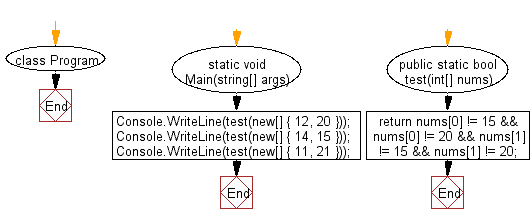﻿ C# - Check if an array of length 2, not contain 15 and 20# C# Sharp Basic Algorithm Exercises: Check whether a given array of integers of length 2, does not contain 15 or 20

## C# Sharp Basic Algorithm: Exercise-98 with Solution

Write a C# Sharp program to check whether a given array of integers of length 2, does not contain 15 and 20.

Pictorial Presentation:Sample Solution:-

C# Sharp Code:

``````using System;
namespace exercises
{
class Program
{
static void Main(string[] args)
{
Console.WriteLine(test(new[] { 12, 20 }));
Console.WriteLine(test(new[] { 14, 15 }));
Console.WriteLine(test(new[] { 11, 21 }));

}
public static bool test(int[] nums)
{
return nums != 15 && nums != 20 && nums != 15 && nums != 20;
}
}
}
```
```

Sample Output:

```False
False
True```

Flowchart:C# Sharp Code Editor:

Improve this sample solution and post your code through Disqus

What is the difficulty level of this exercise?

Test your Programming skills with w3resource's quiz.

﻿